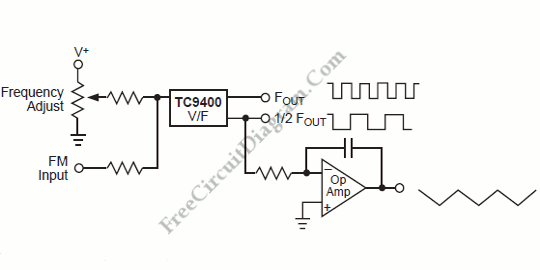## Long Period SCR Timer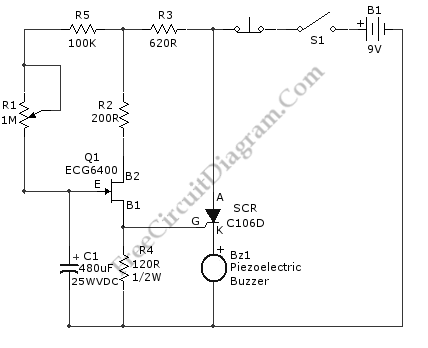This is a SCR timer circuit. Here, SCR is used to drive the final actuator, the buzzer.  The time constanst of this circuit is determined by  R1 and C1. The buzzer will sound when C1 charges up to a desired level, it will cause the Q1 to conduct and triggers SCR1.  The use of FET for Q1 make the RC […]

## Norton Amplifier with DC Gain Control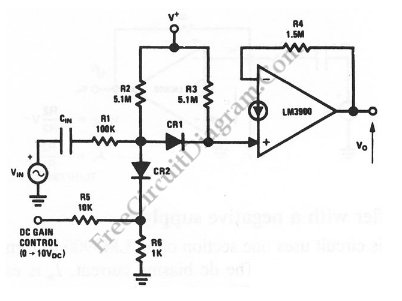This is a Norton amplifier circuit with dc gain control. This circuit is formed by one section of an LM3900. This circuit has dc gain-control input ranges from 10 V for maximum gain down to 0 V for minimum gain. Here is the schematic diagram of the circuit:

## 20 kHz Astable Multivibrator with Transistors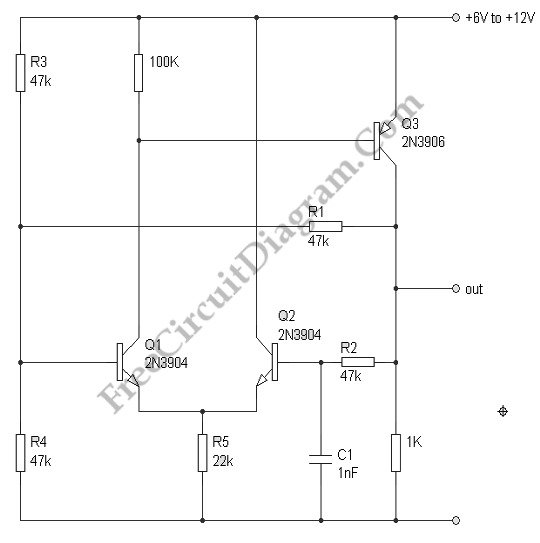Astable multivibrator or oscillator circuit is based on positive feedback. We can design such circuit using op-amp, logic gates, or transistors. Here we can rely on single capacitor capacitor and resistor for the feedback inside op-amp like transistor circuit, which works over wide range of temperature, voltages and transistor gains. The stability is pretty good, for supply voltage changes between […]

## Logarithmic Amplifier Using MC1556 Op-Amp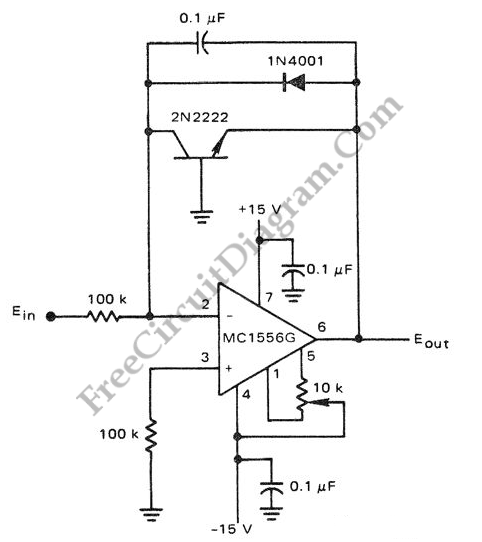The circuit below is a logarithmic amplifier using an MC1556 op amp. The 10K pot is an offset adjustment.  Here is  a schematic diagram of one classic example of  general purpose  logarithmic converter circuit employing LM1556 op-amp: The popularity of logarithmic amplifier is decreasing as the microprocessor and microcontroller chips are  getting lower and lower in price. Long time ago, […]

## 2-MHz Frequency Standard With Dividers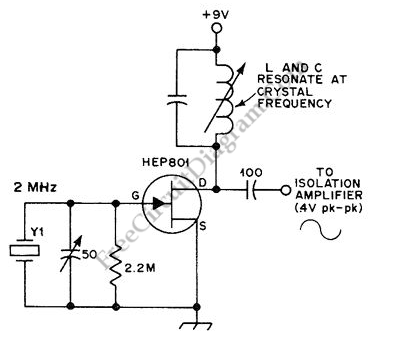This is an oscillator circuit that can be used for calibrating frequency counters, amateur receivers and frequency meters. This circuit is called 2MHz standard with dividers circuit. This circuit uses two crystals, 100kHz and 2 MHz.  Here is the schematic diagram of the oscillator part of the circuit: This circuit is then can be combined with two 7490 decade counter […]

## One Second Steps 555 Timer Circuit (0 To 59 S)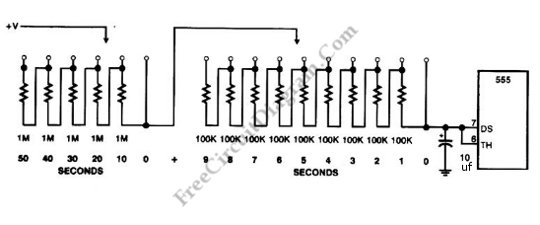The linearity of  555 IC is sufficient to make 1 second precision, and this circuit employs the feature in this design. This timer step circuit can generate a timing steps from 0 to 59 s in 1-s intervals. Here is the schematic diagram of the circuit :